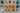# python-custom-sort-function

Tue Jul 21 2020This tiny post is for me, as I was had two chanlleges I did that required "custom sort function". It was super hard to "google" it as things were different in python 2 and I'm using python 3 now.

The bottom line it does the same but just weird sytax.

``````# python 3
from typing import List
from functools import cmp_to_key

weirdList = [
{
"a": 1,
"b": 3,
},
{
"a": 1,
"b": 2,
},
{
"a": 2,
"b": 1,
},
]
def owncomp(a, b):
if a["a"] > b["a"]:
return 1
elif a["a"] < b["a"]:
return -1

if a["b"] > b["b"]:
return 1
else:
return -1

rs = sorted(weirdList, key=cmp_to_key(owncomp))
print(rs)``````

## Simpler Sort Option (only changing key)

``intervals.sort(key=lambda x: x["a"])``